Courses

# Syllogism CLAT Notes | EduRev

## LR : Syllogism CLAT Notes | EduRev

The document Syllogism CLAT Notes | EduRev is a part of the LR Course Logical Reasoning for CLAT.
All you need of LR at this link: LR

Syllogism

First of all you should know some terms as follows:-

Subject and Predicate

For example: Some ships are cargos. Therefore, in this statement ship is the subject and cargos are the predicate.

Identify the type A, E, I, O

A          Affirmative Positive

E          Affirmative Negative

I           Particular Positive

O          Particular Negative

 Affirmative Positive (A) All, Every, Each, Everyone, Particular Name, One Affirmative Negative (E) No one, None, Never, Nothing Particular Positive (I) Some, Many, Several, Few, Very few, Most, at least Particular Negative (O) Some-not

UNDERSTANDING SYLLOGISM WITH HELP OF VENN DIAGRAM

Different Types of arguments:

1. Affirmative Positive -A type :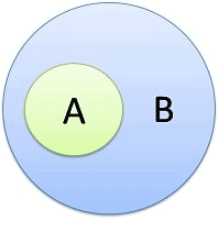Eg. All ‘A’ are ‘B’

From this statement it can be inferred that,

• Some ‘A’ are ‘B’.
• Some ‘B’ are ‘A’ is a definite conclusion.

Note:  All ‘B’ are ‘A’ is a possibility but  it may not be true in all cases

2. Affirmative Negative -E Type :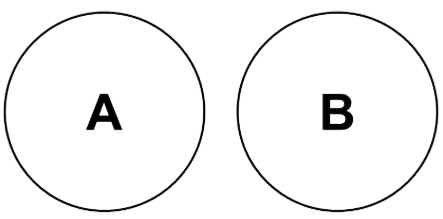Eg. No ‘A’ are ‘B’

Conclusions which can be drawn from given statement are:

• Some ‘A’ are Not ‘B’
• No ‘B’ are ‘A’
• Some ‘B’ are not ‘A’.

3. Particular Positive - I Type :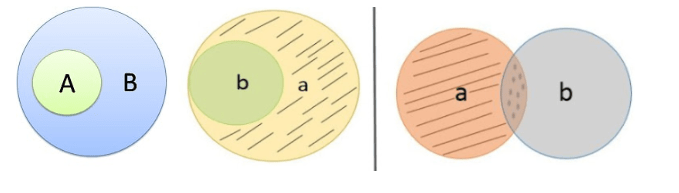Fig (i), (ii) and (iii) respectively

Eg. Some ‘A’ are B’

From given statement we can conclude

• Some ‘B’ are ‘A’. (i)
• However All ‘A’ are ‘B’ (i)
• All ‘B’ are ‘A’, (ii)
• Some ‘A’ are ‘B’ (iii)
• Some ‘A’ are not ‘B’ and Some ‘B’ are not ‘A’ is not a definite conclusion.

4. Particular Negative - O Type :

Eg. Some ‘A’ are not ‘B’.

It cannot be explained with diagram. Moreover no logical conclusion can be drawn from given statement.  There can be more than one possibilities in which this argument can be represented. They are as under: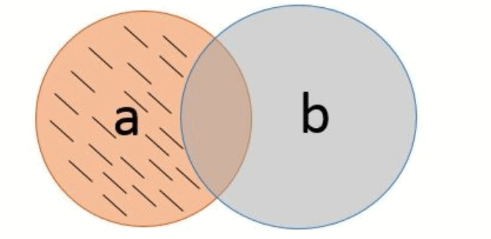The shaded portion shows the A which is not B.

Deriving logical conclusions when various types of arguments are given together:

1. All ‘A’ are ‘B’ ,  All ‘B’ are ‘C’    (A & A)

This argument is represented below.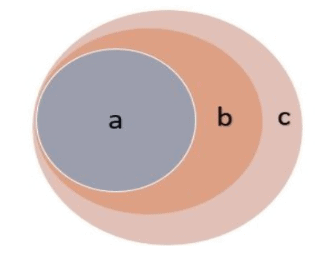Conclusions:

• All ‘A’ are ‘C’ ,
• Some ‘C’ are ‘A’ ,
• Some ‘C’ are ‘B’ ,
• Some ‘A’ are ‘C’
• (All ‘C’ can be ‘A’ , All ‘B’ can be ‘A’ but may not be defiantly true in all the cases.)

2. All ‘A’ are ‘B’ , No ‘B’ are ‘C’  (A & E)

This argument is represented below: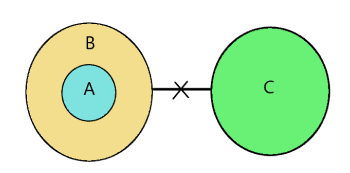• No ‘A’ is ‘C’ ,
• Some ‘A’ are not ‘C’ ,
• Some ‘C’ are not ‘A’ ,
• All ‘A’ are ‘B’.

3. Some ‘A’ are ‘B’ , All ‘B’ are ‘C’ (I & A)

This argument can be  represented by Figure 9 as well as figure 10.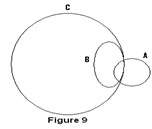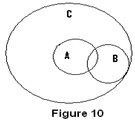Conclusions which are definitely true in both the cases:

• Some ‘A’ are ‘C’,
• Some ‘C’ are ‘A’,
• Some ‘B’ are ‘A’.

Note:      All ‘A’ are ‘C’ , Some ‘A’ are not ‘C’ , Some ‘A’ are not ‘B’ and vice versa can be a possibility but may not be true in all the cases. The other possibility is as under:

1. Some ‘A’ are ‘B’ , No ‘B’ are ‘C’ (I & E)

This argument can be represented by Figure 11, 12 & 13.

Conclusions which are definitely true in all the possible cases:

Some ‘A’ are not ‘C’, Some ‘B’ are not ‘C’, No ‘C’ is ‘B’, Some ‘C’ are not ‘B’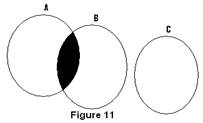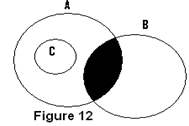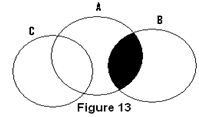The shaded portion shows A which are not C.

Note:      Any conclusion with ‘C’ as subject and ‘A’ as predicate is not a definite conclusion.

1. No ‘A’ are ‘B’ , All ‘B’ are ‘C’ (E & A)

This argument can be represented by Figure 14, 15, & 16.

Conclusions which are definitely true in all the cases:

Some ‘C’ are not ‘A’, No ‘B’ is ‘A’, Some ‘B’ are not ‘A’, Some ‘A’ are not ‘B’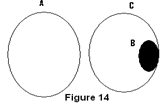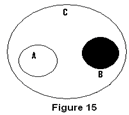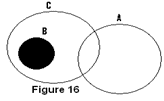The shaded portion shows C which are not A.

Note: Any conclusion with ‘A’ as subject and ‘C’ as predicate is not a definite conclusion.

1. No ‘A’ are ‘B’ , Some ‘B’ are ‘C’ (E & I)

This argument can be represented by Figure 17, 18 & 19.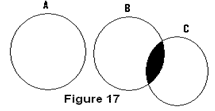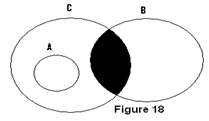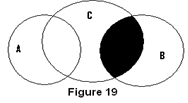Conclusions which are definitely possible in all the cases:  Some ‘C’ are not ‘A’, Some ‘A’ are not ‘B’, Some ‘B’ not ‘A’

Note:  Any conclusion with ‘A’ as subject and ‘C’ as predicate is not a definite conclusion.

Offer running on EduRev: Apply code STAYHOME200 to get INR 200 off on our premium plan EduRev Infinity!

15 docs|54 tests

,

,

,

,

,

,

,

,

,

,

,

,

,

,

,

,

,

,

,

,

,

;